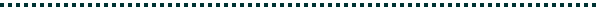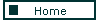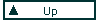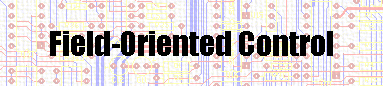Introduction

In spite of the performance improvements that better step-motor models and control algorithms have brought in the last five years, open-loop control falls significantly short of achieving maximum motor performance. Open-loop performance is limited by lack of control of the torque angle of the motor.

Step-motors are permanent-magnet synchronous motors with many poles and some reluctance torque. From basic motor theory, when the stator current (MMF) vector is maintained at a phase angle of 90 degrees ahead of the rotor flux vector, maximum torque is produced. The torque varies with the sine of this torque angle between vectors. By sensing rotor angle, the position and phase of the rotor flux can be determined and the current excitation kept 90 degrees (electrical) ahead of it. At this optimal torque angle, maximum motor torque is available, under both steady-state and transient operation, and is the essence of vector or field-oriented control. The result is behavior like that of a dc motor, but without brush limitations.

Open-loop step-motor control schemes couple path (motion profile) control with motor control by scheduling steps in accordance with the velocity profile. Profiles are modified to minimize jerk, and mechanical load changes often force reduction of maximum torque to maintain path control. The result is an underutilized motor with sensitivity to noise and load variation.

Field-Oriented Control

To overcome open-loop limitations, decouple path from motor control by making the step-motor respond to torque commands from the path controller as a dc motor would. Industrial step-motor applications often use shaft encoders, but they are not used to control torque angle, thus limiting performance. Position feedback is required to control or verify path motion, and this same feedback can be used by the motor controller to control torque angle.

An existing controller implementation for a two-phase step- motor is largely digital, with position input from an incremental encoder attached to the motor shaft. A digital integrator synthesizes a noninteger multiple of the encoder pulse frequency, which drives a ROM-based waveform generator.

The ROM contains both phases of drive current, in quadrature, and alternate phases are output on alternate clock pulses. Each encoder pulse represents a quantum of position, and these pulses are accumulated by an encoder interface and then transferred to the integrand register of the accumulator. The synthesizer clock, fV, is the carry output of the accumulator adder. During each sample interval of the encoder, the position value in the F register is added to the phase accumulator (ACC) at the rate fC/D. F contains the value:

F = fin×Dt = fin/(fC/N)

where fin is the encoder pulse rate and Dt the update period of F. The overflow rate is then,

fV = (F×2-M)×(fC/D)

where M is the number of bits in length of the accumulator, which holds the fractional part of the phase, while the overflow is the integer carry. By substitution for F,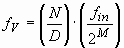and the scaled fin is multiplied by the ratio N/D, which can be a noninteger fraction.

The waveform (sine) ROM output is the electrical waveform at the electrical frequency fel of the motor, and is related to the encoder frequency by substituting for fV in:

fel = fV/2R

Then fel is eliminated and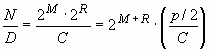For a 2000 count/rev (500 line/rev) encoder, a 50 pole step- motor, M = 5 (4 bits + sign) and R = 8, then N/D = 204.8 and fV = (12.8)fin. The maximum value of F for a maximum speed of 40 rev/s (2000 steps/s) is 11. Also, N = 2048 and D = 10.

To achieve field orientation, the encoder must be aligned on the rotor so that the encoder Z (home) pulse resets the waveform ROM phase address to zero at the rotor position of zero electrical phase. Then at power-up, the motor is stepped until the Z pulse occurs, aligning the electrical phase as to its corresponding ROM address. For each revolution of the encoder, Z ensures that alignment is maintained.

The magnitude of the stator current vector is controlled directly by an analog torque input command. The dual DAC that outputs the winding-phase currents multiplies this command to the digitally generated sinewaves, thus controlling their amplitude. This controller achieved essentially maximum theoretical acceleration of maximum motor torque over rotor inertia.

Winding-Sensed Field-Oriented Control

The rotor alignment, while sensitive, need be done once (at the factory). But for applications not requiring a position encoder for path control, rotor position can be derived instead from the motor induced voltages. A winding-sensed analog implementation uses current-source drives to the windings so that motor induced voltages can be sensed across them. These voltages are integrated to produce representations of the flux waveforms. The integration not only removes high-frequency noise from the PWM of the driver, but keeps amplitude constant with motor speed. By use of the trigonometric identity for sin(ab), where a is the flux angle and b the stator current angle from the waveform ROM, a phase error is developed. This error drives a VCO through a loop compensator; the VCO in turn drives the waveform ROM counter. The phase detector is implemented with a dual multiplying DAC, where sin(b) is the digital input and sin(a) is the reference input.

Use of an analog VCO is low-cost, but of limited frequency range, with a non-zero minimum frequency. A numerically- controlled oscillator (NCO), which is essentially the same as the digital synthesizer of the first scheme, can work down to dc but is more costly to implement. Consequently, a two-mode approach was taken. For motor speeds below that which produces a reliable flux signal and also below that corresponding to the minimum VCO frequency, the controller operates in low-speed mode. At low speeds, the motor is microstepped by the host processor, by providing pulses to the waveform ROM counter that would otherwise come from the VCO. For the low-speed range (from stall to about 50 rpm), dynamic response is generally not critical. Outside this range, the VCO is switched in as the frequency source, and it will have locked in phase at a lower speed before switchover. A commanded torque replaces the microstep pulse as input to the controller outside the low-speed region.

## Closure

For dynamically sensitive step-motor applications, field-oriented control is preferable to microstepping and eliminates the familiar resonance anomalies and torque variations of step- motors. The resulting performance advantages over microstepping compensate for the additional circuit cost and complexity. Either analog or digital implementation is suitable for integration as a mixed-signal IC, which would allow field-oriented step-motor control to be cost-competitive with microstepping.